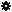﻿ Object Model: Geometry.GetClosestLocations

Geometry.GetClosestLocations

## Geometry.GetClosestLocationsv5.0

### Description

Returns a PointLocatorData object containing the closest surface locations from the specified input positions. By default, input positions have to be defined in the object's local space reference.

Some aspects of the closest locations computation such as the reference pose or the method (closest surface, closest vertex or knot, ...) can be set up using Geometry.SetupPointLocatorQueries. This setup will affect all subsequent calls to GetClosestLocations and to Geometry.GetClosestLocationsWithinRadius.

When you call this method an acceleration cache is automatically created. See Geometry.SetupPointLocatorQueries for more information.

Notice that the "closest distance" relationship may change relative to the spatial reference of the geometry and the input positions. See Geometry.SetupPointLocatorQueries for more information.

Notice that the returned point locators can be evaluated on any Geometry instance having the same topology.

### C# Syntax

 ```PointLocatorData Geometry.GetClosestLocations( Object in_pPositions ); ```

### Scripting Syntax

 ```oReturn = Geometry.GetClosestLocations( Positions ); ```

### Parameters

Parameter Type Description
Positions Array An array of Doubles, either formatted as a 1D array of packed XYZ triplets, or as a 2D array of XYZ triplets.

### Examples

#### 1. JScript Example

 ```/* Example using PointLocatorData to find the closest mesh sphere vertex from a Null */ NewScene(); var NullObj = GetPrim("Null", null, null, null); Translate(NullObj, -2.0, 8.0, 5.0, siRelative, siView, siObj, siXYZ); var SphereGeom = CreatePrim("Sphere", "MeshSurface").ActivePrimitive.Geometry; var ClosestPointLocator = SphereGeom.GetClosestLocations(NullObj.Kinematics.Global.Transform.GetTranslationValues2()); var ClosestPosition = SphereGeom.EvaluatePositions(ClosestPointLocator).toArray(); var TriangleVertices = SphereGeom.GetTriangleVertexIndexArray(ClosestPointLocator).toArray(); var TriangleWeights = SphereGeom.GetTriangleWeightArray(ClosestPointLocator).toArray(); Application.LogMessage("The closest position on the mesh sphere from the Null is (" + ClosestPosition + ", " + ClosestPosition + ", " + ClosestPosition + ")"); Application.LogMessage("which corresponds to the triangle made of vertices (" + TriangleVertices + ", " + TriangleVertices + ", " + TriangleVertices + ")."); Application.LogMessage("The barycentric weight relatively to each triangle vertex is (" + TriangleWeights + ", " + TriangleWeights + ", " + TriangleWeights + ")."); //INFO : The closest position on the mesh sphere from the Null is (-0.6959691047668459, 3.2883720624894605, 1.8517908803876053) //INFO : which corresponds to the triangle made of vertices (14, 21, 22). //INFO : The barycentric weight relatively to each triangle vertex is (0.34798455238342285, 0.12156937271356582, 0.5304460525512695). ```

#### 2. JScript Example

 ```/* This example uses PointLocatorData to shrink-wrap a sphere to a cube, and then push the sphere along the cube's normals. */ NewScene(null, false); var root = Application.ActiveSceneRoot; var CubeGeom = root.AddGeometry("Cube", "MeshSurface").ActivePrimitive.Geometry; var SphereObj = root.AddGeometry("Sphere", "MeshSurface"); SphereObj.subdivv = 24; SphereObj.subdivu = 24; // We must freeze it, otherwise its position array cannot be set: FreezeObj(SphereObj); var SphereGeom = SphereObj.ActivePrimitive.Geometry; var SphereOnCubePointLocators = CubeGeom.GetClosestLocations(SphereGeom.Points.PositionArray); var SphereOnCubePositions = CubeGeom.EvaluatePositions(SphereOnCubePointLocators).toArray(); var SphereOnCubeNormals = CubeGeom.EvaluateNormals(SphereOnCubePointLocators).toArray(); for (i = 0; i < SphereOnCubePositions.length; i++) SphereOnCubePositions[i] += SphereOnCubeNormals[i]*3; SphereGeom.Points.PositionArray = SphereOnCubePositions; ```